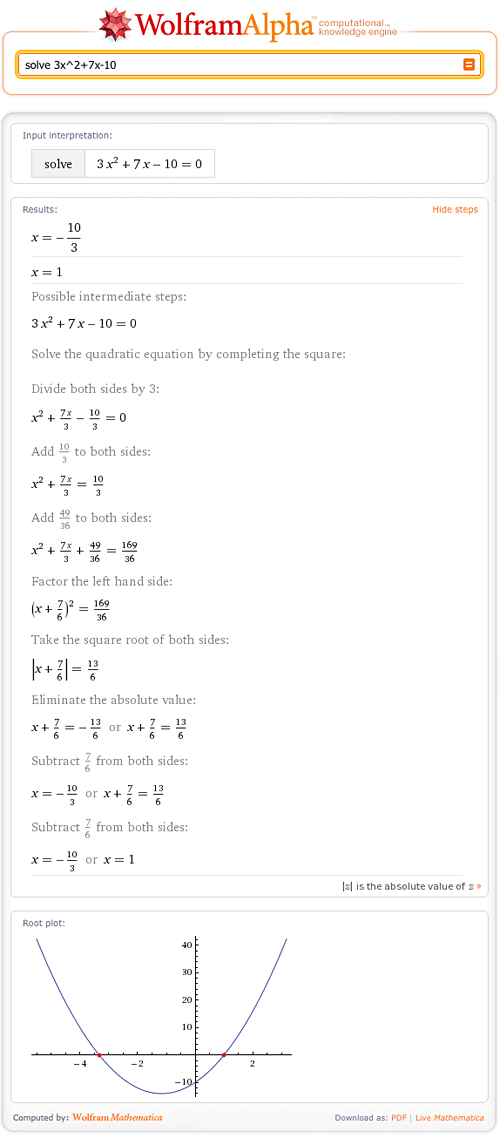TAG: Fractions
September 2, 2010–

A new school year is here, and many students are diving into new levels of math. Fortunately, this year, you have Wolfram|Alpha to help you work through math problems and understand new concepts. Wolfram|Alpha contains information from the most basic math problems to advanced and even research-level mathematics. If you are not yet aware of Wolfram|Alpha’s math capabilities, you are about to have a “wow” moment. For the Wolfram|Alpha veterans, we have added many math features since the end of the last school year. In this post, we’re highlighting some existing Wolfram|Alpha math essentials, such as adding fractions, solving equations, statistics, and examples from new topics areas like cusps and corners, stationary points, asymptotes, and geometry.

You can access the computational power of Wolfram|Alpha through the free website, via Wolfram|Alpha Widgets, with the Wolfram|Alpha App for iPhone, iPod touch, and the iPad! Even better, the Wolfram|Alpha Apps for iPhone, and iPod touch, and the iPad are now on sale in the App Store for \$0.99 though September 12.

If you need to brush up on adding fractions, solving equations, or finding a derivative, Wolfram|Alpha is the place to go. Wolfram|Alpha not only has the ability to find the solutions to these math problems, but also to show one way of reaching the solution with the “Show Steps” button. Check out the post “Step-by-Step Math” for more on this feature.You can find this widget, and many others, in the Wolfram|Alpha Widget Gallery. Customize or build your own to help you work through common math problems. Then add these widgets to your website or blog, and share them with friends on Facebook and other social networks.

Of course, Wolfram|Alpha also covers statistics and probability. For example, Wolfram|Alpha can compute coin tossing probabilities such as “probability of 21 coin tosses“, and provides information on normal distribution: More »

December 1, 2009–

## July 17, 2019 Update: Step-by-step solutions has been updated! Learn more.

Have you ever given up working on a math problem because you couldn’t figure out the next step? Wolfram|Alpha can guide you step by step through the process of solving many mathematical problems, from solving a simple quadratic equation to taking the integral of a complex function.

When trying to find the roots of 3x2+x–7=4x, Wolfram|Alpha can break down the steps for you if you click the “Show steps” button in the Result pod.As you can see, Wolfram|Alpha can find the roots of quadratic equations. Wolfram|Alpha shows how to solve this equation by completing the square and then solving for x. Of course, there are other ways to solve this problem! More »